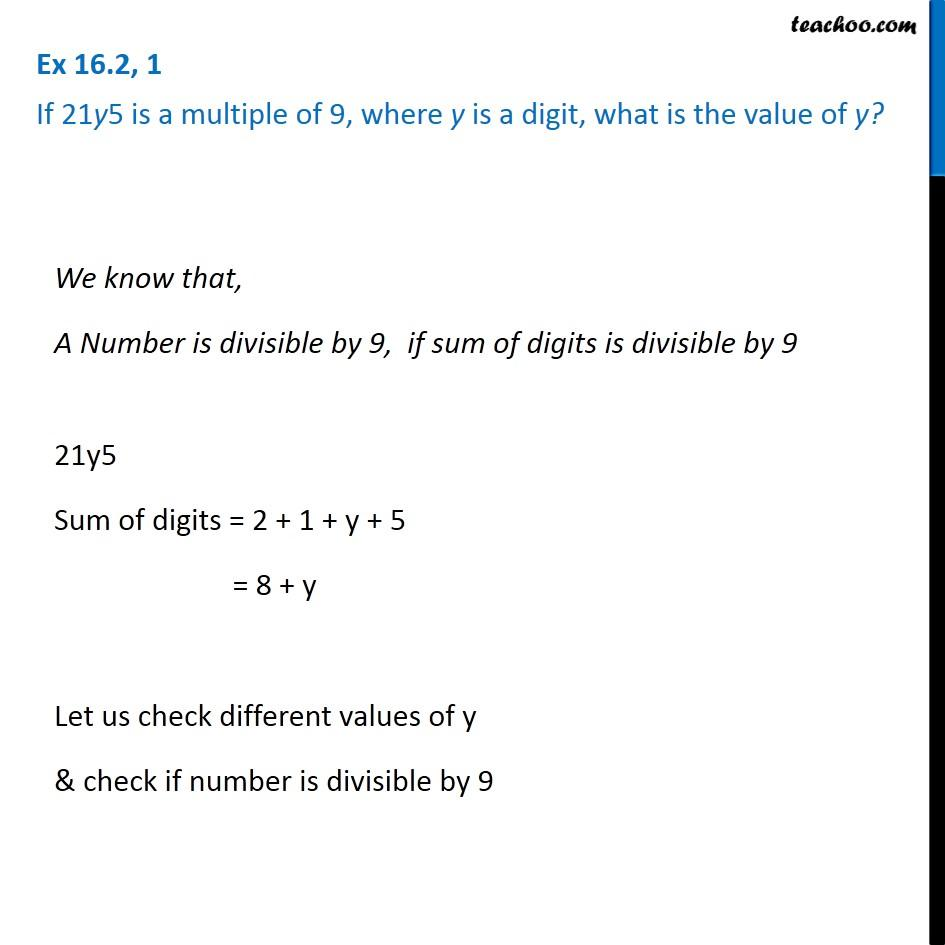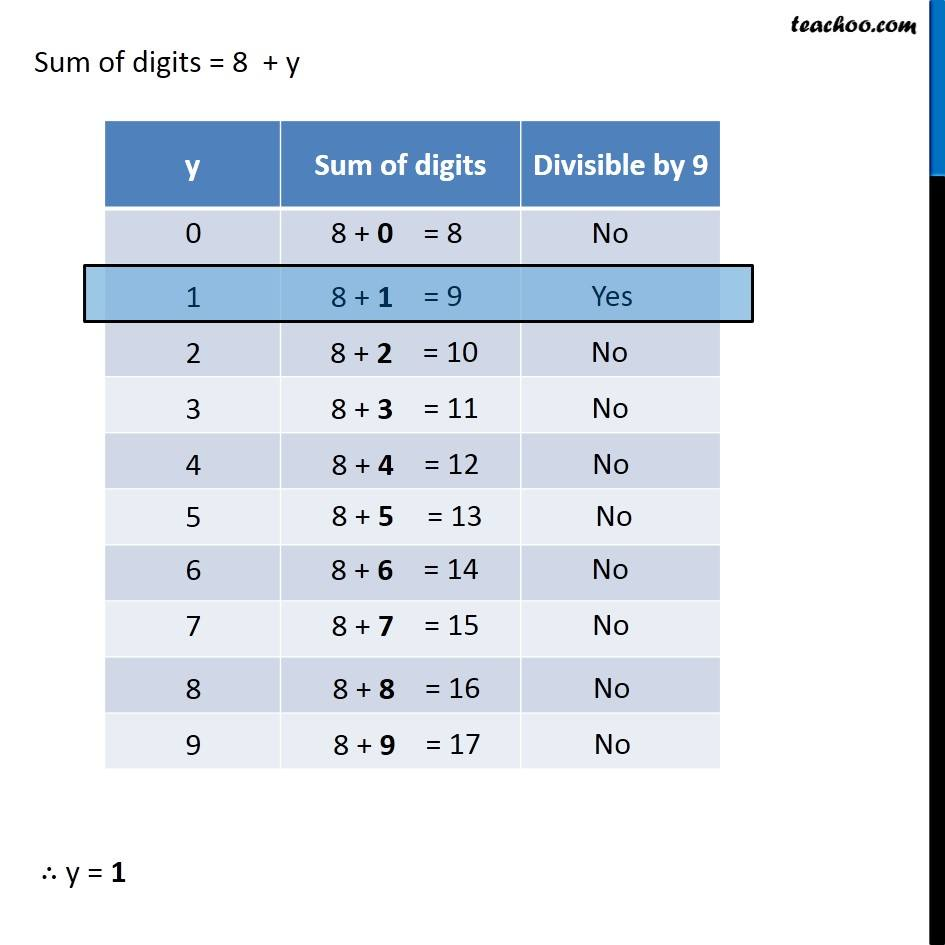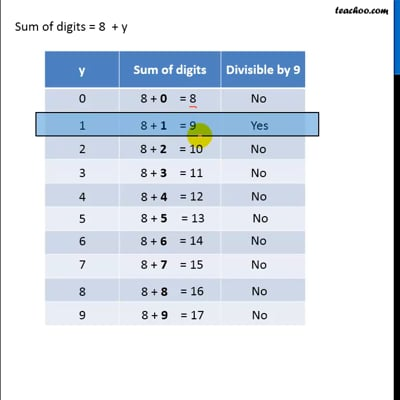Ex 16.2

Chapter 16 Class 8 Playing with Numbers
Serial order wiseThis video is only available for Teachoo black users

### Transcript

Ex 16.2, 1 If 21y5 is a multiple of 9, where y is a digit, what is the value of y? We know that, A Number is divisible by 9, if sum of digits is divisible by 9 21y5 Sum of digits = 2 + 1 + y + 5 = 8 + y Let us check different values of y & check if number is divisible by 9 Sum of digits = 8 + y